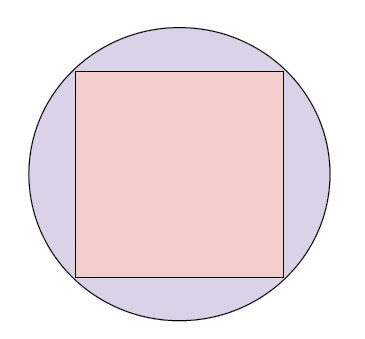# Area of a Circumscribed Circle of a Square in C++

In this problem, we will calculate the area of the circumscribed circle of a square when we are given the side of the square. Before we go further let’s revise the basic definitions to understand the concepts better.

Square is a quadrilateral with all sides equal.

The circumscribing circle is a circle touches all the vertices of a polygon.

The area is the quantitative representation of the extent of any two-dimensional figure.

To calculate the area of the circumscribed circle of a square. We need to find the relation between the parameter of the circle and the square.Now, as in the figure, all the vertices of the square are touching the circle. We can conclude from seeing the figure that the diagonal of the square is equal to the diameter of the circle.

Using this we can derive the relationship between the diameter of the circle and side of the square.

r = (√ (2a^2))/2

r is the radius of the circle and the side of the square.

Now, using the formula we can find the area of the circle.

Area of circle = π*r^2
= π* ((√ (2a^2))^2 / 2
= π * (2 *a ^ 2)/4
= (π*a^2)/2

Now, using this formula we can find the area of the circle.

## Algorithm

Step 1 : Calculate area of circle using formula {(3.14 * a * a) /2 }
Step 2 : Print the area of the circle

## Example

Live Demo

#include <iostream>
using namespace std;
int main(){
float a = 6;
float area = ( (3.14 * a * a )/2) ;
cout<<"The area of Circumscribed Circle of a Square of side "<<a<<" is "<<area;
return 0;
}

## Output

The area of Circumscribed Circle of a Square of side 6 is 56.52